# Practice questions for electromagnetic Induction

## Paragraph Based Questions

(A) A metal jumper of mass m can slide without friction along two parallel metal guides directed at an angle ? and separated by the distance a. The guides are connected at the bottom through an uncharged capacitor of capacitance C.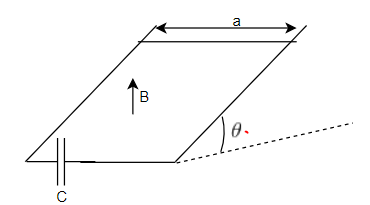The entire system is placed in an upward magnetic field of induction B
At the initial moment ,the jumper is held at a distance b from the foot of the hump

Question 1
What is the acceleration of the jumper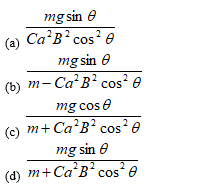Question 2
What is the time taken to reach bottom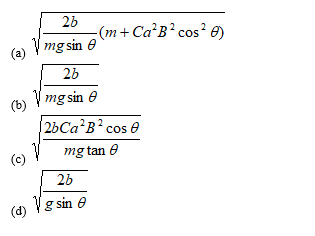Question 3
Find the instantaneous EMF as a function of time t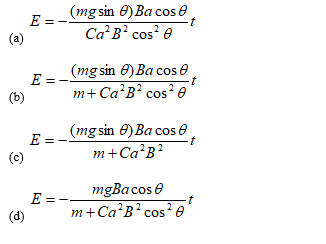Question 4
What will be the velocity at the bottom
(a)$\sqrt {\frac {2bmg \sin \theta}{m + Ca^2B^2 \cos ^2 \theta}}$
(b)$\sqrt {\frac {2bmg \cos \theta}{m + Ca^2B^2 \cos ^2 \theta}}$
(c)$\sqrt {\frac {2amg}{m + Ca^2B^2 \cos ^2 \theta}}$
(d)$\sqrt {\frac {2bmg}{m + Ca^2B^2 \cos ^2 \theta}}$

(B) A square loop of wire of side (b) lies on table ,at a distance s from a very long straight wire which carries a current I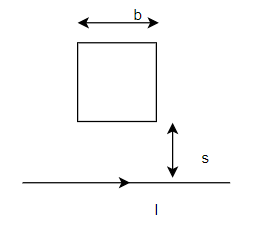Question 5
Find the flux of magnetic field through the loop
(a) $\frac{\mu_0Ib}{4\pi}\frac{s+b}{s}$
(b) $\frac{\mu_0Ib}{2\pi}(\frac{s+b}{s})$
(c) $\frac{\mu_0Ib}{2\pi}ln{\frac{s}{s+b}}$
(d) $\frac{\mu_0Ib}{2\pi}ln{\frac{s+b}{s}}$

Question 6
If the loop is now pulled away from wire at a constant speed ,what emf will be generated in the loop
(a) $\frac{\mu_0Ib^2v}{2\pi}$
(b) $\frac{\mu_0Ib^2v}{2\pi bs}$
(c) $\frac{\mu_0Ib^2v}{2\pi b(s+b)}$
(d) $\frac{\mu_0Ib^2v}{2\pi b(s-b)}$

Question 7
What if the loop is pulled to right at speed v ,instead of away. What is the induced EMF
(a) $\frac{\mu_0Ib^2v}{2\pi bs}$
(b) $\frac{\mu_0Ib^2v}{2\pi b(s-b)}$
(c) 0
(d) $\frac{\mu_0Ib^2v}{2\pi b(s+b)}$

## Multiple Choice Questions

Question 8
The rectangular loop shown in below figure moves towards the origin at a velocity
$v=-250 \mathbf{j}$ m/s
There exists a Magnetic field in the region such that
$B=.80e^{-.50y}\mathbf{k}$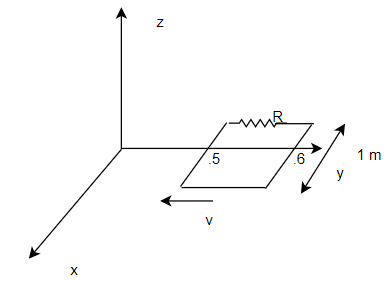Find the current at the instant the coil sides are at y=.50m and .60m.
The value of R =2.5 ohm
(a) 3.04A
(b)2 A
(c)3 A
(d) 0

Question 9
A square loop of side L ,Mass M and total resistance R falls vertically into uniform magnetic field B directed perpendicular to the plane of the coil.The height h through which the loop falls so that it attain terminal velocity on entering the region of magnetic field
(a) $\frac{MgR^2}{4B^3L^3}$
(b) $\frac{M^2gR^2}{2B^4L^4}$
(c) $\frac{M^2gR^2}{2B^2L^2}$
(d) $\frac{MgR}{2BL}$

## Paragraph Based Questions

(C) A metal disk (radius =b and thickness =w) is placed in a solenoid with its axis coincident with the axis of the solenoid. The solenoid produces a magnetic field
$B=B_0sin{2}\pi ft$
Consider a small dotted ring of radius r as shown in fig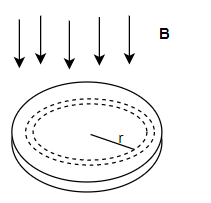Question 10
calculate the EMF induced in the small ring
(a) $2\pi^2r^2fB_0sin{2}\pi ft$
(b) $2\pi r^2fB_0cos{2}\pi ft$
(c) $2\pi^2r^2B_0cos{2}\pi ft$
(d) $2\pi^2r^2fB_0cos{2}\pi ft$

Question 11
If $\rho$ is the resistivity of the material and width of the ring is dr, what is the value of eddy current in the dotted ring
(a) $\frac{\pi f B_0wrdr}{\rho}$
(b) $\frac{\pi f B_0w(sin{2}\pi ft)rdr}{\rho}$
(c) $\frac{fB_0w(cos{2}\pi ft)rdr}{\rho\pi}$
(d) $\frac{\pi f B_0w(cos{2}\pi ft)rdr}{\rho}$

Question 12
Find the total power loss due to eddy current in the disks
(a) $\frac{\pi^3wb^4f^2}{2\rho}{cos}^2{2}\pi ft$
(b) $\frac{\pi^3wb^4f^2}{\rho}{cos}^2{2}\pi ft$
(c) $\frac{\pi^3wf^2}{2\rho}{cos}^2{2}\pi ft$
(d) $\frac{\pi^3wb^4f^2}{2\rho}$

(D) A long solenoid of Radius r has n turns and it carries an alternating current
$I=I_0sin{\omega}t$
A concentric circular wire of Radius R( R> r) surrounds the solenoid

Question 13
Find the induced EMF generated in the wire
(a) $\mu_0\pi\omega nI_0r^2cos{\omega}t$
(b) $\mu_0\pi\omega nI_0R^2cos{\omega}t$
(c) $\mu_0\pi\omega nI_0R^2sin{\omega}t$
(d) $\mu_0\pi\omega nI_0(R-r)^2cos{\omega}t$

Question 14
Find the induced Electric field at any point inside the wire
(a) $\frac{\mu_0\omega n I_0R^2cos{\omega}t}{2r}$
(b) $\frac{\mu_0\omega n I_0r^2cos{\omega}t}{R}$
(c) $\frac{\mu_0\omega n I_0r^2sin{\omega}t}{2R}$
(d) $\frac{\mu_0\omega n I_0r^2cos{\omega}t}{2R}$

(E)A rod AB of length L is placed along x -axis and it is pivoted at origin O such that A(-a,0) and B(b,0) . The magnetic field in the region is
$B=-B_0\mathbf{k}$
The rod is rotated in the x -y plane around the z axis with angular velocity $\omega$ in anti clockwise direction
Question 15
Find the polarity of the ends A and B
(a) Both are positive
(b) Both are negative
(c) A is positive and B is negative
(d) A is negative and B is positive
Question 16
Find the induced EMF between Ab
(a) $V_A-V_B=B_0\omega(b^2-a^2)$
(b) $V_A-V_B=\frac{1}{2}B_0\omega(a^2-b^2)$
(c) $V_A-V_B=\frac{1}{2}B_0\omega(b^2-a^2)$
(d) None of these

(F)A square loop side a and resistance R lies a at distance a from infinte straight wire that carries current I. The current varies as
$I(t)=(1-\alpha t)$ for 0$\le t\le1/\alpha$
$I(t)=0$ for $t>1/\alpha$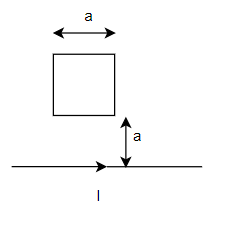Question 17
Find the induced EMF in the loop
(a) $\frac{\mu_0a}{2\pi}\alpha$
(b) $\frac{\mu_0aln{2}}{\pi}\alpha$
(c) $\frac{\mu_0aln{a}}{2\pi}\alpha$
(d) $\frac{\mu_0aln{2}}{2\pi}\alpha$
Question 18
Find the induced current in the loop
(a) $\frac{\mu_0a\alpha}{2\pi R}$
(b) $\frac{\mu_0a\alpha l n{a}}{2\pi R}$
(c) $\frac{\mu_0a\alpha l n{2}}{\pi R}$
(d) $\frac{\mu_0a\alpha l n{2}}{2\pi R}$
Question 19
what total charge passes a given point in the loop during the time current flows
(a) $\frac{\mu_0aln{2}}{\pi R}$
(b) $\frac{\mu_0aln{4}}{2\pi R}$
(c) $\frac{\mu_0aln{2}}{2\pi R}$
(d) $\frac{\mu_0aln{2}}{R}$

(G) Conductors are joined together to form a circuit as shown below in figure.The resistance of a unit length of conductor is r.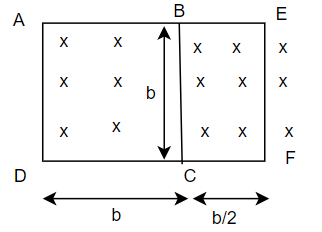The arrangement is placed in a magnetic field which is perpendicular to the plane of the circuit. And acting downward
The Magnetic field is varying with time as per the below expression
$B=B_0t$
Question 20
Find the current in the AD branch
(a) $\frac{3B_0b}{11r}$
(b) $\frac{7B_0b}{11r}$
(c) $\frac{B_0b}{22r}$
(d) $\frac{7B_0b}{22r}$
Question 21
Find the current in the BC branch
(a) $\frac{3B_0b}{11r}$
(b) $\frac{7B_0b}{11r}$
(c) $\frac{B_0b}{22r}$
(d) $\frac{7B_0b}{22r}$
Question 22
Find the current in the EF branch
(a) $\frac{3B_0b}{11r}$
(b) $\frac{7B_0b}{11r}$
(c) $\frac{B_0b}{22r}$
(d) $\frac{7B_0b}{22r}$

## Multiple Choice questions

Question 23
Two concentric coplanar circular loops made of wire with resistance per unit length $10^{-4}$ O/m have diameters .2 m and 2 m respectively.
A time varying potential difference $V=(4+2.5 t)$ V is applied to larger loop.
Find the induced current in the small loop
(a) 3 A
(b) 2 A
(c) 1 A
(d) 1.25 A

Question 24
A coil PQR of Radius 'a' carries a current I and it is placed on the XY plane with origin as center .A small conducting ring ABC of radius b ( a> >>b) is also XY plane with center at $z=z_0$. The ring ABC is allowed to fall free over the ring PQR with velocity v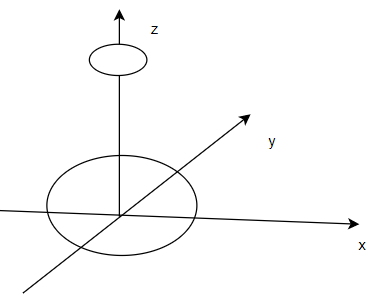Find the induced EMF in the ring ABC as function of z
(a) $\frac{3\mu_0\pi I a^2b^2zv}{2(a^2+z^2)^{5/2}}$
(b) $\frac{3\mu_0\pi I a^2b^2zv}{2(a^2+z^2)^{3/2}}$
(c) $\frac{3\mu_0\pi I a^2zv}{2(a^2+z^2)^{5/2}}$
(d) $\frac{3\mu_0\pi I b^2zv}{2(a^2+z^2)^{5/2}}$

Question 25
A wire frame of Area S and resistance R is suspended freely from a thread of length L. There is a uniform magnetic field $B_0$ existing in space and plane of the wire frame is perpendicular to the magnetic field. The wire frame is made to oscillate under the force of gravity by displacing it through distance A from it initial position along the direction of the magnetic field.It is given A <<< Find the induced EMF in the wire frame as a function of time.
(a) $\frac{BSA^2\omega}{L^2}sin{2}\omega t$
(b) $\frac{BSA^2\omega}{2L^2}cos{\omega}t$
(c) $\frac{BSA^2\omega}{2L^2}sin{2}\omega t$
(d) None of these

Question 26
A ring of a rectangular cross-section is made of a material whose resistivity is $\rho$. The ring is placed in a magnetic field . The magnetic field is directed along the axis of the ring and increase directly with time
$B=B_0t$.
Find the induced current in the ring
(a) $\frac{B_0h}{4\rho}b^2$
(b) $\frac{B_0h}{2\rho}(b^2-a^2)$
(c) $\frac{B_0hab}{4\rho}$
(d) $\frac{B_0h}{4\rho}(b^2-a^2)$

Question 27
A rectangular loop is being pulled at a constant speed v, through a region of certain thickness d, in which a uniform magnetic field B is set up. The graph between position x of the right hand edge of the loop and the induced emf E will be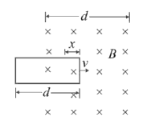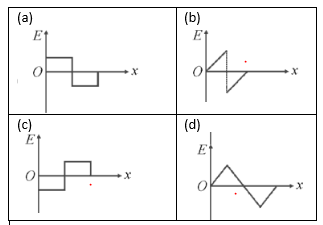Question 28
A conducting square loop of side l and resistance R moves in its plane with a uniform velocity v perpendicular to one of its sides. A magnetic induction B constant in time and space, pointing perpendicular and into the plane at the loop exists everywhere with half the loop outside the field, as shown in figure. The induced e.m.f. is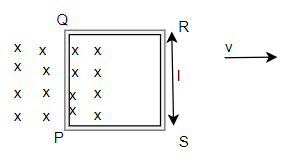(a) vlB
(b) vlB/2
(c) 2vlB
(d) zero

Question 29
The coxial cable has an inner wire of Radius $R_1$ and an outer metal sheath with inner radius radius $R_2$ .The current I flows down the inner wire and back through the sheath.
What is the self inductance of the unit length of the cable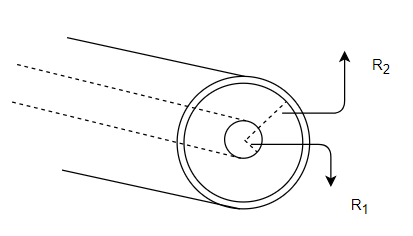(a) $\frac{\mu_0}{2\pi}\frac{R_2}{R_1}$
(b) $\frac{\mu_0}{2\pi}\frac{R_1}{R_2}$
(c) $\frac{\mu_0}{2\pi}ln{\frac{R_2}{R_1}}$
(d) None of these

Question 30
Two neighboring coils X and Y have have 300 and 600 turns respectively .
It is find that the current 1.5 A in X causes $1.2 \times 10^{-4}$ wb to pass in X and $.9 \times 10^{-4}$ wb through Y
Which of the following is correct
(a) Mutual inductance of the coils X and Y is 36 mH
(b) Self inductance of the coil X is 24 mH
(c) Self inductance of the coil Y is 24 mH
(d) none of these

Question 31
A long solenoid X of diameter .1 m has $2 \times 10^4$ turns per meter .At the center of the solenoid a 100 turn coil Y of radius .01 m is placed with its axis coinciding with the solenoid axis. The current in the solenoid is decreased at a constant rate from +2A to -2A in .05 sec.The resistance of the coil is $10\pi^2\Omega$
Which of the following is true
(a) The EMF induced in the coil is $6.3 \times 10^{-2}$V
(b) The mutual inductance of the system is H
(c) The current flowing in the coil is $5 \times 10^{-4}$ A
(d) The flux in the small coil at current 2A is Weber

Question 32
The mutual inductance between the rectangular loop and long straight wire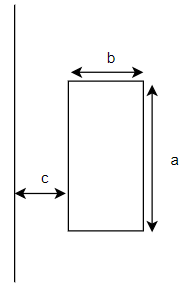(a) $\frac{\mu_0a}{2\pi}ln{(}1+\frac{b}{c})$
(b) $\frac{\mu_0a}{2\pi}ln{(}1+\frac{c}{b})$
(c) $\frac{\mu_0a}{2\pi}ln{\frac{b}{c}}$
(d) $\frac{\mu_0a}{2\pi}ln{\frac{c}{b}}$

Question 33
A wire in the form of a circular loop of Diameter D lies with its plane normal to magnetic field B. The wire is pulled to take a equilateral shape in same plane in 1 sec.Find the Emf induced in the loop
(a) $E=\frac{B\pi D^2}{4}\left(1-\frac{\pi}{4}\right)$
(b) $E=\frac{B\pi D^2}{4}\left(1-\frac{\pi\sqrt3}{4}\right)$
(c) $E=\frac{B\pi D^2}{4}\left(1-\frac{\pi\sqrt3}{2}\right)$
(d) $E=\frac{B\pi D^2}{4}\left(1-\frac{\pi\sqrt3}{8}\right)$

Question 34
A circular coil of Diameter D of metal wire is kept stationary in a non uniform magnetic field given by
$\mathbf{B}=B_x\mathbf{i}+B_y\mathbf{j}+B_z\mathbf{k}$
Let E and I be the Induced Emf and current in the coil
Statement 1: $E \ne 0$ ,$I \ne 0$
Statement 2: E=0 ,I=0
Statement 3: $E \ne 0$ , I=0
Statement 4: E=0 , $I \ne 0$

Which of the following is true
(a) Statement 2 is true
(b) Statement 3 is true
(c) statement 1 is true
(d) statement 4 is true

An alternating current flows down a straight wire which runs along the axis of a toroidal coil with rectangular crosssection of inner radii a ,outer radii b ,height h and N turns) The coil is connected to the R ohm resistor
Question 35
What is the EMF induced in the torroid coil
(a) $\frac{\mu_0Nh\omega}{2\pi}I_0sin{\omega}t$
(b) $\frac{\mu_0Nh\omega}{2\pi}ln{\left(\frac{b}{a}\right)}I_0cos{\omega}t$
(c) $\frac{\mu_0Nh\omega}{2\pi}ln{\left(\frac{a}{b}\right)}I_0sin{\omega}t$
(d) $\frac{\mu_0Nh\omega}{2\pi}ln{\left(\frac{b}{a}\right)}I_0sin{\omega}t$

Question 36
Find the Self Inductance of the toriod
(a) $L=\frac{\mu_0N^2h}{2\pi}ln{\frac{b}{a}}$
(b) $L=\frac{\mu_0N^2h}{2\pi}ln{\frac{q}{b}}$
(c) $L=\frac{\mu_0N^2h}{2\pi}$
(d) $L=\frac{\mu_0N^2}{2\pi}ln{\frac{b}{a}}$

Question 37
Find the back EMF induced in the torriod due to changing current in the wire
(a) $\frac{\mu_0^2N^3h^2\omega I_0}{4\pi^2R}\left(ln{\frac{b}{a}}\right)^2cos{\omega}t$
(b) $\frac{\mu_0^2N^3h^2I_0}{4\pi^2R}\left(ln{\frac{b}{a}}\right)^2sin{\omega}t$
(c) $\frac{\mu_0^2N^3h^2\omega I_0}{4\pi^2R}\left(ln{\frac{b}{a}}\right)^2sin{\omega}t$
(d) $\frac{\mu_0^2N^3h^2\omega I_0}{4\pi^2}\left(ln{\frac{b}{a}}\right)^2sin{\omega}t$

## Multiple Choice Questions

Question 38
An e.m.f is produced in a coil, which is not connected to an external voltage source. This can be due to
(a) the coil being in a time varying magnetic field.
(b) the coil moving in a time varying magnetic field.
(c) the coil moving in a constant magnetic field.
(d) the coil is stationary in external spatially varying magnetic field, which does not change with time

Question 39
A coil of wire having finite inductance and resistance has a conducting ring placed coaxially within it. The coil is connected to a battery at time t = 0, so that a time-dependent current $I_1(t)$ starts flowing through the coil. If $I_2(t)$ is the current induced in the ring. and B(t) is the magnetic field at the axis of the coil due to $I_1(t)$, then as a function of time (t > 0), the product $I_2 (t) B(t)$
(a) Increases with time
(b) Decreases with time
(c) Does not vary with time
(d) Passes through a maximum

Question 40
Two circular coils P & Q are fixed coaxially & carry currents $I_1$ and $I_2$ respectively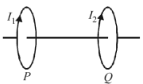(a) if $I_2$= 0 & P moves towards Q, a current in the same direction as $I_1$ is induced in Q
(b) if $I_1$= 0 & Q moves towards P, a current in the opposite direction to that of $I_2$ is induced in P.
(c) when $I_1 \ne 0$ and $I_2 \ne 0$ are in the same direction then the two coils tend to move apart .
(d) when $I_1 \ne 0$ and $I_2 \ne 0$ are in opposite directions then the coils tends to move apart.